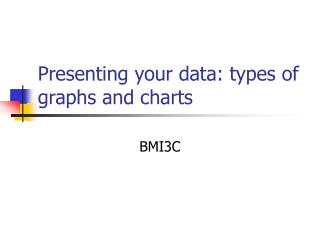DownloadDownload PresentationPresenting your data: types of graphs and charts

# Presenting your data: types of graphs and charts

Télécharger la présentation## Presenting your data: types of graphs and charts

- - - - - - - - - - - - - - - - - - - - - - - - - - - E N D - - - - - - - - - - - - - - - - - - - - - - - - - - -
##### Presentation Transcript

1. Basic Types of Graphs • There are many types of graphs, but these are the most common: • Pie Chart • Bar Graph • Line Graph • The type of graph you choose depends mostly on the type of data you have

2. Pie Graph • What portion of the total does this part make up? • A pie graph allows us to compare parts of the whole with each other, or the fraction of the whole each part takes up

3. Pie Graph • Let's say you eat half of the cake - how much of the cake is left? • Obviously, if you ate one side of the cake, then the other side is still there • But now say you weren't quite so hungry, so you only ate half of that half - how much is left of the cake now? • The most you could ever possibly eat is the whole cake (100%), because after that, there's no more cake left! The less you eat of the cake, the more you have left over.

4. Pie Graph • What are some statistics that OTHS could use that would best be presented in Pie Graph form?

5. Bar Graphs • How different are these variables from each other? • Bar graphs are great for looking at differences amongst similar things

6. Bar Graphs • Let's say we wanted to look at the number of marine mammals sighted • We wanted to know the least seen and the most seen marine mammals out on the water • Bar graphs are great because you can stack numbers of things right next to each other and compare instantly • The height of each stack can tell you the number of marine mammals, either approximately by the numbers on the vertical axis or exactly by labeling each stack with the number • Bar graphs are good for giving a comparison of absolute numbers.  However, if we wanted to compare what portion each stack may represent of all the marine mammals combined, we need a pie graph.

7. Bar Graphs • What are some statistics that OTHS could use that would best be presented in Bar Graphs form?

8. Line Graphs • How does this variable change over time? • Basically, plotting dots on a chart showing the different values over time and connecting the dots to create a line

9. Line Graphs • Let's say we have data on dolphin sightings for a particular area. • The data tells us the date and the number of dolphins seen. • First, let's make the dots.  We would move horizontally along the graph with the dates in order, and then go up vertically to the number of dolphins sighted for each date. • Now we connect the dots (by connecting the dots we suggest that the data points are related) • We can see how the numbers change over time – for example we can see seasonal changes in the dolphin population

10. Line Graphs • What are some statistics that OTHS could use that would best be presented in Line Graphs form?

11. Which type of graph? • the number of female students in each grade in your school • the annual number of road fatalities (the road toll) in your province or territory over the last 30 years • the speed (km/h) of the world's 20 fastest animals • the total population of Canada, the provinces and territories